# Projective object of a category

(diff) ← Older revision | Latest revision (diff) | Newer revision → (diff)
A concept formalizing a property of retracts (or direct summands) of free groups, free modules, etc. An objectof a categoryis said to be projective if for every epimorphism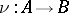and every morphismthere is a morphism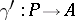such that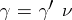. In other words, an objectis projective if the representable functorfrom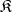to the category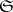of sets takes epimorphisms ofto epimorphisms of, i.e. to surjective mappings.
Examples. 1) In the category of sets every object is projective. 2) In the category of groups, only free groups are projective. 3) In the category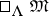of left modules over an associative ring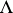with a unit, a module is projective if and only if it is a direct summand of a free module. The description of the rings over which every projective module is free constitutes the content of the Serre problem. 4) In the categoryall modules are projective if and only if the ringis classically semi-simple. 5) In the category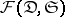of functions from a small category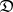to the categoryof sets, every object is projective if and only ifis a discrete category.
In the definition of projective objects it is sometimes supposed that the functor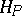takes not all the epimorphisms but only the morphisms of a distinguished class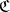to surjective mappings. In particular, ifis the class of admissible epimorphisms of a bicategory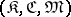, thenis called an admissible projective object. For instance, in some group varieties, the free groups of that variety are admissible projective objects with respect to the class of all surjective homomorphisms but are not projective objects since there exist non-surjective epimorphisms.